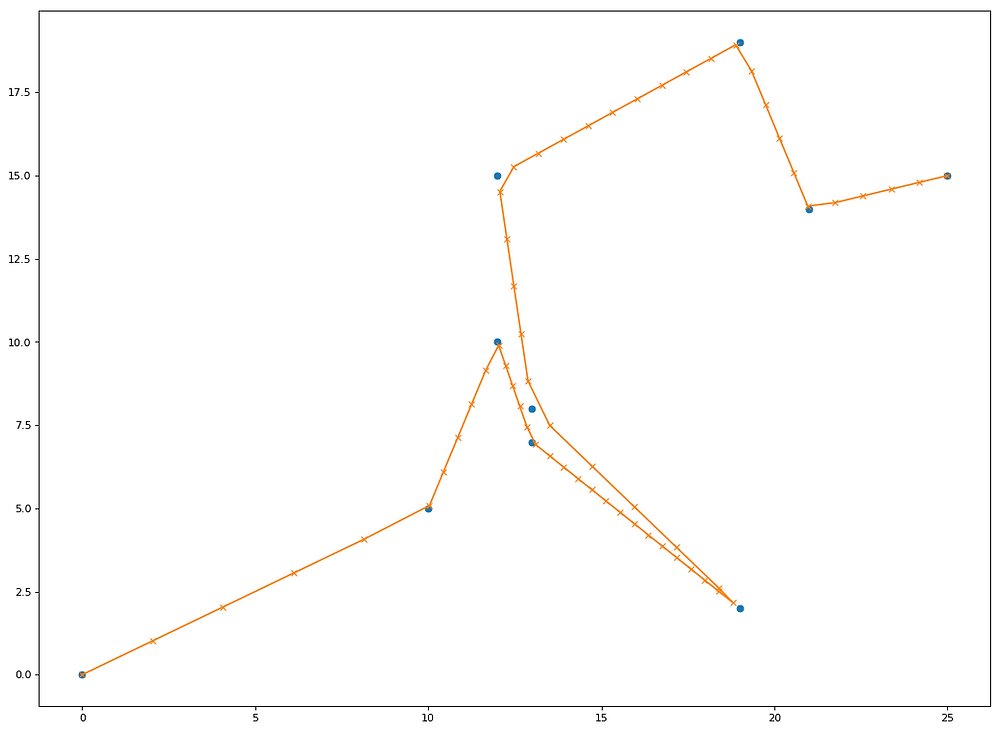Search
• Andrew Jones

# Linear Interpolation (Lerping) in Python

Linear Interpolation is a method of curve fitting using linear polynomials to construct new data points within the range of a discrete set of known data points.

In practice, this could mean you can infer new, estimated location points between known location points to either create higher frequency data, or to fill in missing values.

In it's simplest form, visualise the below image:Here, the known data points are in red, at position (1,1) and (3,3). Using linear iterpolation, we can add a point between them, which can be seen in blue.

That was a very simple problem, so what if we had more known data points, and wanted a specific frequency of interpolated points?

This can be achieved quite simply in Python using two functions from the numpy package.

Example 1:

Let's create ten x and y values that follow a sine curve

import numpy as np import matplotlib.pyplot as plt

x = np.linspace(0, 2*np.pi, 10) y = np.sin(x)

plt.plot(x, y, 'o') plt.show()We have ten known points, but let's say we want a sequence of 50 instead.

We can do this with np.linspace, providing; the start point for the sequence, the end point for the sequence, and the number of data points we want in total

The starting point and end point will be the same as the starting and end points for your initial x values, so here we specify 0 and 2*pi. We also specify our request for 50 data points in the sequence

xvals = np.linspace(0, 2*np.pi, 50)

Now, to the linear interpolation! Using np.interp, we pass the list of required data points (the 50 we created above) and then our original x and y values

yinterp = np.interp(xvals, x, y)

Now, let's plot our original values and then overlay our new interpolated values!

plt.plot(x, y, 'o') plt.plot(xvals, yinterp, '-x') plt.show()Example 2:

You could also apply this logic to x and y coordinates in a time-series. Here you would interpolate the x values with respect to time, and then the y values with respect to time. This would be particularly useful if you wanted more frequent data points in your time-series (perhaps you wanted to overlay some data over the frames of a video) or if you were missing data points or had inconsistent timestamps.

Let's create some data for a scenario in which, over a 60 second race lap, a racing car only emitted ten positional (x & y) outputs (there were also at inconsistent times throughout the 60 second period):

import numpy as np import matplotlib.pyplot as plt

timestamp = (0,5,10,15,30,35,40,50,55,60) x_coords = (0,10,12,13,19,13,12,19,21,25) y_coords = (0,5,10,7,2,8,15,19,14,15)

Now, let's create the start time for the race, the end time, and the duration - we'll need these values for the linear interpolation

start_timestamp = min(timestamp) end_timestamp = max(timestamp) duration_seconds = (end_timestamp - start_timestamp)

Apply the spacing, and the the interpolation independently for the x values and the y values

new_intervals = np.linspace(start_timestamp, end_timestamp, duration_seconds)

new_x_coords = np.interp(new_intervals, timestamp, x_coords) new_y_coords = np.interp(new_intervals, timestamp, y_coords)

Let's have a look at the interpolated positional values that we now have:

plt.plot(x_coords, y_coords, 'o') plt.plot(new_x_coords, new_y_coords, '-x') plt.show()Hopefully, this has been useful!